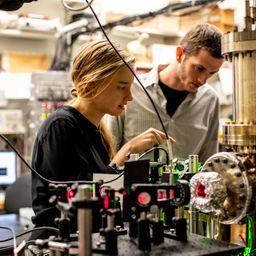Ask your homework questions to teachers and professors, meet other students, and be entered to win \$600 or an Xbox Series X 🎉Join our Discord!Numerade Educator

# Find the speed of an electron having a de Broglie wavelength equal to its Compton wavelength. Hint: This electron is relativistic.

## v=\frac{\sqrt{2} c}{2}

Quantum Physics

Atomic Physics

### Discussion

You must be signed in to discuss.
##### Top Physics 103 Educators##### Andy C.

University of Michigan - Ann Arbor##### Aspen F.

University of Sheffield##### Jared E.

University of Winnipeg### Video Transcript

Okay. So for this one, we want to find the speed of an electron having a competent. It's comfortably blank, people to is to brutally with flying. So Compton wavelength is cool. T h over m c. So why let Liam to see Is each over m c? And I'm sorry, Is that one of his age? Yes, over embassy. And then we want to set it equal to the de Brookly way. Playing took probably wavelength, which is people t h o ver p. And then we want to use relativistic mo mentum. So I think you just end up putting a gamma in front of it. I'm gonna pause the video. Well, I look it up. No, you just put a game in front. So, um and then what's cama? Uh, Gammas. So we have m v. And then gamma is the square root of one who was at minus her. Plus, don't forget that one minus b squared over speaks part. I don't know of a great way to remember it, but I guess if I I do relativity, we'll probably find a nice way to remember it. Royalty Vitti chapter. Okay, so now we want to solve for this vys. That's gonna be kind of analogy. Break mess. Hopefully, it's not too bad. Let's start by canceling those. Let's get some stuff out of the denominator by cross multiplying. Oops. I should have canceled that sorry over here, so it didn't really make sense to cancel that. So and then Oh, actually, this is kind of nice. The masses also cancel. So, basically, now, let's go ahead and move Everything, um, elsewhere. So So I'm gonna bring the sea over here, so C is equal to V over the square root of one minus V squared over C squared. That's gonna interesting. Really Doesn't depend on in any physical constants. Um, double tracking The entrepreneur looks good to me. Now we need to solve for V. All right, so here's where the algebra mess comes so we can square. Both sides might try to take some shortcuts to avoid, like, tons of different lines. Well, that was seamless. Okay. And then let's multiply both sides by one minus. V squared over C squared. So bring this over here. Actually, this could be kind of fun. Thio, uh, try to do all the Al Jeroen online so I multiply both sides by that great and then oh yes, it's really not so bad. So let's distribute the C squared and I will do any line for that. C squared minus B squared equals b squared. I could bring the B square to the other side so adding plus V squared to both sides when it's that makes this to be square and then great. And now I end up with us such a simple result that V is equal to the square root, um of 1/2 just multiplied by C. That was fun. So I guess it has to be basically on part of the speed of light for the confidently like equal to its superbly with thanks.University of Washington

#### Topics

Quantum Physics

Atomic Physics

##### Top Physics 103 Educators##### Andy C.

University of Michigan - Ann Arbor##### Aspen F.

University of Sheffield##### Jared E.

University of Winnipeg